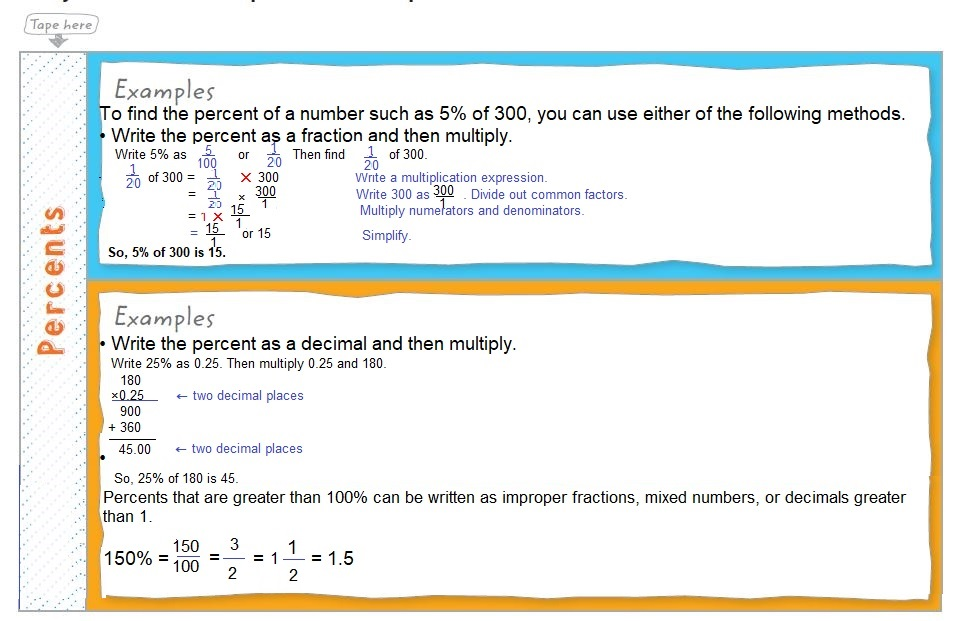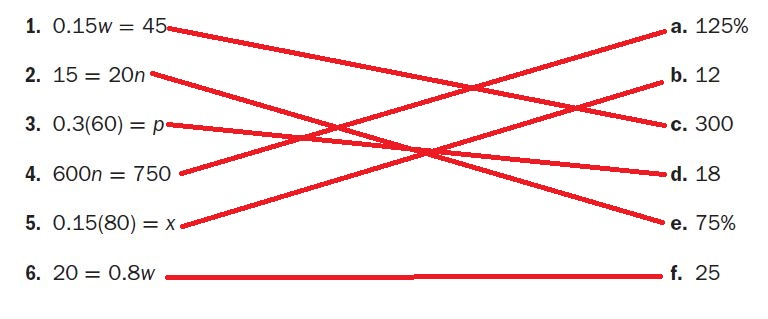Homework Explained - Math Practice 101Dear guest, you are not a registered member. As a guest, you only have read-only access to our books, tests and other practice materials.

As a registered member you can:

• View all solutions for free
• Request more in-depth explanations for free
• Ask our tutors any math-related question for free
• Email your homework to your parent or tutor for free
Registration is free and doesn't require any type of payment information. Click here to Register.
Go to page:
Grade 7 McGraw Hill Glencoe - Answer Keys
Chapter 2: Percents; Chapter Review

Please share this page with your friends on FaceBookUse your Foldable to help review the chapter.• Question 1

Got it?
Match each equation with its solution.0.15w = 45

•  $$1:$$
•  $$2:$$
• Question 3

0.3(60) = p

•  $$3:$$
•  $$4:$$
• Question 5

0.15(80) = x

•  $$5:$$
•  $$6:$$

Yes, email page to my online tutor. (if you didn't add a tutor yet, you can add one here)